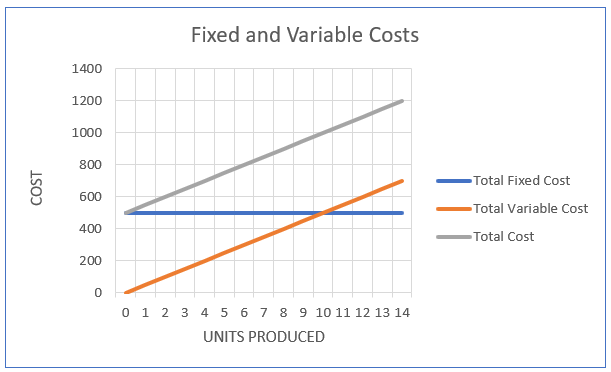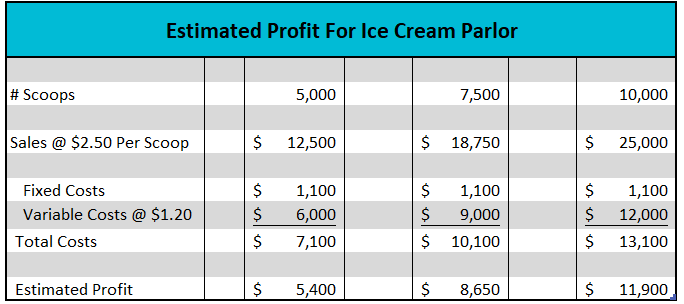# Fixed Cost

## Definition of Fixed Cost:

Fixed costs are costs that are not directly influenced by how much of a good or service is produced. Examples of fixed costs include rent, mortgage payments, telephone bills, and salaries of essential personnel.

## Detailed Explanation:

The Fixed and Variable Costs graph below illustrates a business’s cost structure. The blue line, which represents the company's fixed costs, is horizontal because it is unaffected by output. It intersects the y-axis at the total fixed cost because fixed costs must be paid even when there is no production. In the illustrated example, the fixed cost equals \$500, and the company would incur a \$500 cost if production dropped to zero (such as when a manufacturing plant closes for a week in the summer), and remains at \$500 if production increases to 14 units. The orange line represents the business's variable costs. Variable costs are expressed on a per-unit basis. On the graph it is assumed that the variable cost per unit remains constant, so the total variable cost (TVC) equals the variable cost per unit (VC) times the number of units produced (Q). TVC = VC (Q). The variable cost graph originates at the origin and has a positive slope equal to the variable cost per unit. The formula for total costs is fixed costs plus the total variable cost (FC + TVC), where FC is fixed costs. The total cost curve is the sum of the variable and fixed cost curves.How many scoops would you need to sell to cover your fixed and variable expenses? In business, this is known as your break-even. Every scoop generates \$1.30 in revenues that can be used to pay your fixed costs (Price – VC). If your fixed costs total \$1,100, you would need to sell 847 scoops to cover your fixed costs or break-even. The break-even formula is:

Break-Even # Units = FC / (Price - VC)

Break-Even # Units = \$1,100 / \$2.50 - \$1.20

Fixed costs have a direct impact on a company's break-even. If the fixed costs increases, more of a good or service would need to be produced to achieve break-even, assuming there is no change in the variable cost or price.

## Dig Deeper With These Free Lessons:

Output and Profit Maximization
Fundamental Economic Assumptions
Factors of Production – The Required Inputs of Every Business
Marginal Analysis – How Decisions Are Made